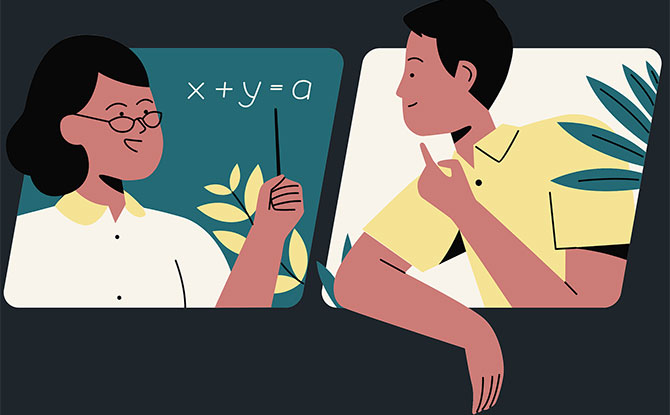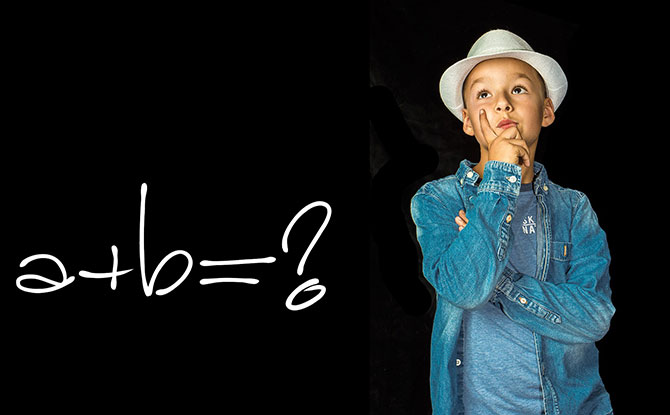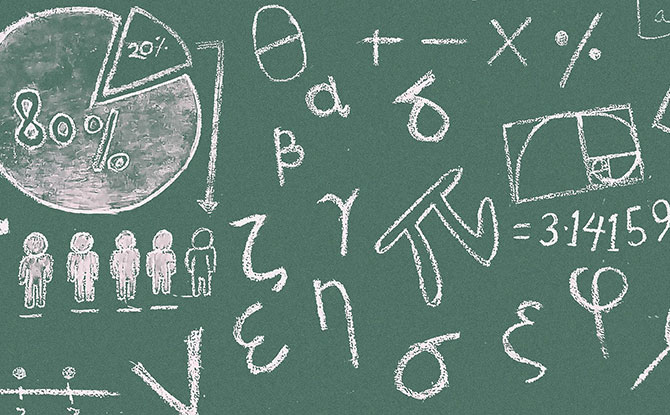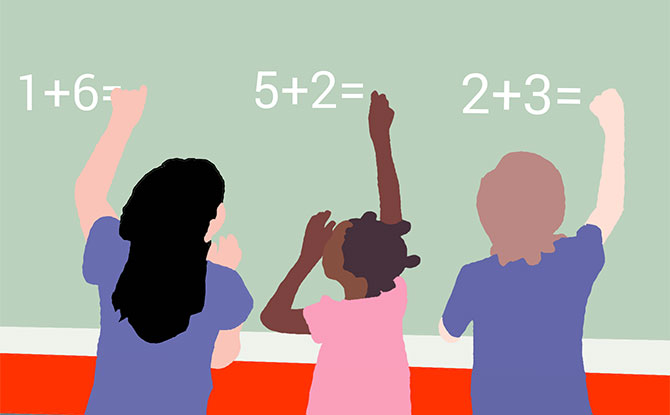# 120 Math Jokes & Puns To Get You Laughing On A TangentSharing is caring!

Math jokes are fun. They can help to add a bit of levity into a dreary class and spice up the formulas on the chalkboard. Math is often seen as a dry and boring subject, but it can actually be quite fun! The key to enjoying math is to find the right approach for you.  Whether you are looking for algebraic laughs or geometric fun, there are 120 math jokes and puns to tickle your funny bone below.

## Math Jokes, Riddles & Puns

1. Why didn’t the circle want to attend math class?

2. What is the most humble mathematical operator?
The equal sign, because it was neither greater now less than anyone else.

3. Which monster is good at math?
Count Dracula.

### Must-Know June Holiday Camps 2023 In Singapore: Workshops & Programmes For...

4. What did the math teacher plant in his garden?
A geometry.

5. How do math teachers get from Point A to Point B?
Using the rhom-bus.

6. How do you make seven even?
Subtract the “S”.

7. Why was the odd job man fired?
Of the six he was supposed to do, he only did jobs one, three and five.

8. How did the student find a tutor?

9. What is a nocturnal birds’ favorite type of math?
Owl-gebra.

10. What are math teachers’ favorite type of plants?
Plants with square roots.

11. Why was 69 scared of 70?
Because the last time they fought, 71.

12. Why did the chicken cross the Mobius Strip?
To get to the same side.

13. Why couldn’t numbers 5 and 7 find anyone to go on a double date?
Because they were an odd couple.

14. What do you call a chicken that’s good at math?
Mathema-chicken.

15. Why did the math teacher have a long face?
Because he had a lot of problems.

16. What do you call two Ls?
A parallel.

17. Where do math teachers go to dance?
The square dance.

18. What do you call a pair of dudes that love math?
Algebros.

19. How do ghosts solve a quadratic equation?
By completing the scare.

20. What do a football game and a dollar have in common?
Four quarters.

21. What did the mathematician say when he saw the numbers one, three and five?
That’s odd.

22. Why did the statistician only tell an occasional joke?
Because he was an outlier.

23. Why can’t you trust an obtuse angle?
Because they are never right.

24. Why was the snake good at math?

25. What tables don’t you have to learn?
Ping-Pong tables.

26. What is a math teacher’s favorite snake?
A pi-thon.

27. What is three multiplied by twenty, minus thirty-nine?
A math problem.

28. How do you flirt with a mathematician?
With an acute angle.

29. Why did the two fours skip breakfast?

30. Why was the class suspicious of their math teacher when he walked in with graph paper?
Because they knew he was plotting something.

31. What do mathematicians bring on a camping trip?
Axes.

32. Why are decimals so clever?
Because they always have a point.

33. Why should you trust abacuses?
Because you can count on them.

34. Why didn’t the math teacher eat dinner?
Because he could binomials.

35. Why did one-fifth go to the masseuse?
Because it was two-tenths.

## Calculating the Best Silly Math Jokes & PunsSome people love solving puzzles, while others enjoy trying to find patterns. There are even some who simply enjoy the challenge of working through difficult problems. Whichever approach you prefer, there are plenty of ways to make math enjoyable. Just enjoy these funny math jokes – great for both students and teachers alike.

36. Why didn’t the statistician stand out from the crowd?
Because he was average.

37. Why did the trigonometry movie given a PG rating?
Because it was too graphic.

38. Why did the minus sign get a humanitarian award?
For making a difference.

39. What do math students do when it snows?
Make snow angles.

40. Why should you not miss math classes?

41. What do you call a number in a toga?
A roamin’ numeral.

42. What is a mathematician’s favorite dessert?
Pi a la Mode.

43. Why was the circle so popular?
Because it was an all-rounder.

44. What do math teachers like to put in their fireplace?
Natural logs.

45. How did the math student prepare for the geometry lesson?
By exercising. So he could get in shape.

46. Why is the number Pi always lonely at a party?
Because no one want to talk to it as it goes on forever.

47. What do you get when you cross a dog with a calculator?
A friend you can count on.

48. Who is the king of the classroom?
The ruler.

49. What happened to the angle that was caught in a trash compactor?
It became a wrecked-angle.

50. What did the mathematician say when he couldn’t solve the problem?

51. What do you call a mathematician who has just returned from a summer holiday?
A tan gent.

52. How do you solve any equation?
With a pencil.

53. Why was the geometry teacher late for school?

54. Which political party supports mathematician farmers?
Pro-tractors.

55. What did the number two say to the imaginary number?
Get real.

56. Why did the student hand in a blank sheet of paper for his math test?
It was on imaginary numbers.

57. Who was the largest knight at the Round Table?
Sir Cumference.

58. Why couldn’t the angle get a loan?
Because it couldn’t find anyone to cosine.

59. What type of teacher calls his students average?
One that is mean.

60. Why did the mathematician get elected as mayor?
He promised to solve the inequalities.

## Funny Math JokesMath jokes can involve trigonometry puns and geometry riddles. After all, there are so many “angles”, “shapes” and forms that these mathematical jokes can take.

One great way to make math more fun is to incorporate it into games and jokes. There are tons of board games and card games that use basic math skills. Many popular video games also require players to use basic mathematical concepts. Not only is this a great way to practice your math skills, but it can also be a lot of fun!

61. What shape waits for you at Starbucks?
A line.

62. Why can’t a nose be 12 inches long?
Because it would turn into a foot.

63. Why did the math teacher throw the clock out of the window?
He wanted to see time fly.

64. Why didn’t the quarter roll down the hill with the dime?

65. Why was the fraction worried about marrying the decimal?
She was worried about having to convert.

66. Why was algebra easy for the Romans?
Because X was always 10.

67. What did the bee say when it solved the problem?
Hive got it.

68. What do you call a kettle whistling at the top of a mountain?
A high-pot-in-use.

69. What did the student think when the teacher frowned at his trigonometry test?
This isn’t a good sine.

70. Why did the math teacher refuse the drink with eight ice cubes in it?
Because it was too cubed.

71. Why didn’t the math student do the geometry problems?
Because that’s where he drew the line.

72. Why is it sad that parallel lines have so much in common?
Because they will never meet.

73. What is a math teacher’s favourite time of the year?
Sum-mer.

74. Why did the mathematician keep crashing his car?
Because he was a poor deriver.

75. What is 2n plus 2n?
I don’t know, it is 4n to me.

76. Why did the student wear spectacles during math class?
To improve di-vision.

77. Why was the student on the floor doing multiplication problems?
Because his teacher told him not to use tables.

78. Why is a math teacher’s favourite tool?
Multi-pliers.

79. What is a swimmer’s favourite form of math?
Dive-ison.

80. Why did the geometry class take such a long time?
The teacher went off on a tangent.

81. What are ten things that a math teacher can count on?
His fingers.

82. What do you call a math teacher who specialises in algebra?
An X-pert.

83. Why did seven eat nine?
He was trying to make sure he got three square meals a day.

84. What happens when you divide the circumference of a jack-o-lantern by its diameter?
Pumpkin Pi.

85. Why did the math teacher keep spilling his food in the oven?
He was following the instructions, “Put it in the oven at 180 degrees.”

86. What is a butterfly’s favourite subject in school?
Mothematics.

87. What is the most dangerous shape?
A trapezoid.

88. After the sheepdog finished herding all the sheep back into the pen, he reported to the farmer and said, “All 20 sheep accounted for.”

The farmer said, “But I only have 18 sheep.”

The sheepdog replied, “I rounded them up.”

89. Why did the student miss the lesson on circles?
Because there was no point.

90. There are three kinds of people in this world? Those who can count and those who can’t.

91. What type of math are fisherman good at?
Cod-ratic equations.

92. Why should you never start a discussion about infinity?
Because it will never end.

93. Why was the new math teacher hired by the school?

94. What happened to the math teacher who made a miscalculation?
He ate humble pi.

95. What did one math book say to the other math book?
Don’t bother me, I’ve got my own problems.

96. Why doesn’t it make sense for people to be excited about Pi?
Because it is irrational.

97. What did the math teacher say when something went wrong?
It figures.

98. Only a fraction of people can tell the difference between a numerator and a denominator.

99. What’s an opinion without 3.14?
An onion.

## Math Puns and Laughs for a Good TimeCount on having a good time with silly math puns that will create laughter all around. There is plenty of laughs that can be spread with funny math puns and riddles.

100. What do you call a wizard that’s good at math?
A mathemagician.

101. What is a geometry teacher’s favourite sport?
Angling.

102. Why was the fraction not included in the math book?
It was not part of the equation.

103. Why was six terrified of seven?
Because seven, eight, nine.

104. Why wasn’t the student afraid of advanced math?
Because he was told it was as easy as Pi.

105. Why is geometry important?
Without it, life would be pointless.

106. How do deaf mathematicians communicate?
In sine language.

107. Why was the student kicked out of math class?

108. Why don’t mathematicians serve beer at their parties?
Because they can’t drink and derive.

109. Why doesn’t the number four get invited to parties?
Because it is two squared.

110. What happens when you cross a mathematician with a clock?
Arithma-ticks.

111. What do baby parabolas drink?

112. What did the geometry teacher say when his pet parrot escaped?
Oh, no! Polygon.

113. What do parallel lines and vegetarians have in common?
They do not meat.

114. Why should you never argue with a 90-degree angle?
Because they’re always right.

115. Where do math teachers go when in New York City?
Times Square.

116. What shape removes spells and curses?
A hexagon.

117. Why did the triangle join the basketball team?
Because it was good at three-pointers.

118. What happened to the math teacher who afraid of negative numbers?
He stopped at nothing to avoid them.

119. Why did the police arrest the number 11?
It was their prime suspect.

120. Why can’t you trust a mathematician?
Because they are always calculating.

Want more silly jokes? How about some funny elephant jokes?